1594257960

# Address class imbalance easily with Pytorch

In the previous article, we saw how to address class imbalance by oversampling with WeightedRandomSampler. In practice, this reduces risks of overfitting. In this article,** we will show how _WeightedRandomSampler _is implemented and give some intuition to the user.** We first give an example where we apply simple statistics and then we tackle mathematically a more general scenario. The goal is to understand how we end up having the same number of observations from each class even though they are unbalanced.

Nothing worse than not understanding what we use, right? 🤗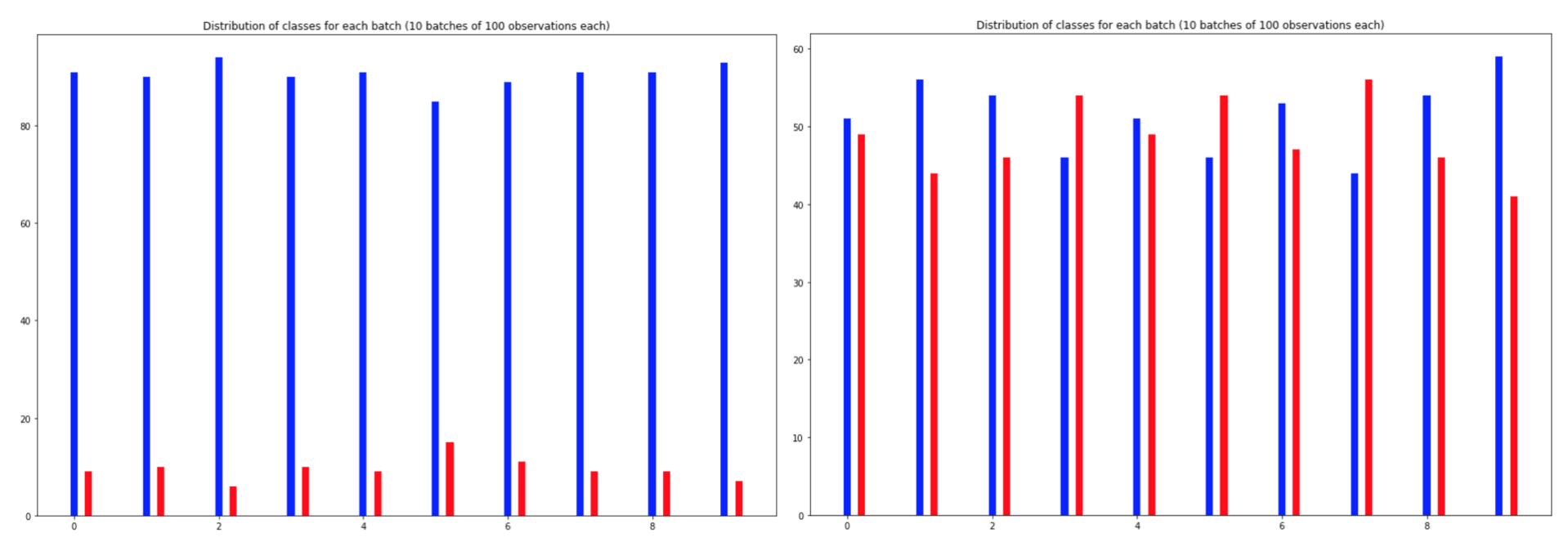Distribution of classes in 10 batches of our dataset. In red we have the minor class and in blue the major class. We go from an unbalanced dataset on the left to a more balanced dataset on the right using WeightedRandomSampler.

Let’s now look at the source code of WeightedRandomSampler implemented in Pytorch. We will do a bit of theory to understand the code and then look at a simple example to get a good grasp of the implementation.

In the source code from Pytorch, the key function is as follows: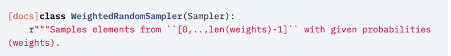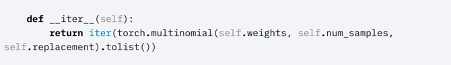In the class WeightedRandomSampler, the key function is call iter.

_A key idea: _drawing from a multinomial distribution with controlled parameters.

Pytorch uses a multinomial distribution with the given parameters, namely the weights, the number of samples and whether we sample with replacement or not.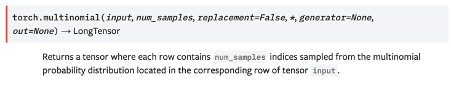The key idea introduced by Pytorch is to draw from a multinomial distribution on the set of points. Each point is assigned a given probability of being sampled. Such probability is defined by its class with the given weight parameter.

## A simple example:

Let us suppose that our data points are ordered as in the following: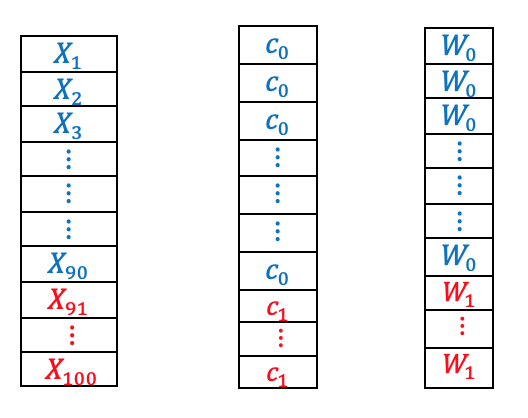100 observations on the left, their class distribution in the center and the weight parameters assigned by WeightedRandomSampler on the right. In blue is represented the major class and in red the minor class.

We can control the weights so that we give** more weight to the minor class**: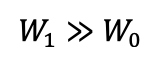We then draw a multinomial distribution with controlled parameters. Each parameter defines the probability of a drawing a given observation. Indeed, we draw from a multinomial distribution over the set of observations.

#pytorch #bayesian-statistics #data-science #machine-learning #data analysis

## Buddha Community1617449307

## CA Classes - Best CA Classes Online

Chartered Accountancy course requires mental focus & discipline, coaching for CA Foundation, CA Inter and CA Finals are omnipresent, and some of the best faculty’s classes have moved online, in this blog, we are going to give the best way to find online videos lectures, various online websites provide the CA lectures, Smartnstudy one of the best site to CA preparation, here all faculty’s video lecture available.

check here : ca classes

#ca classes online #ca classes in delhi #ca classes app #ca pendrive classes #ca google drive classes #best ca classes online1594257960

## Address class imbalance easily with Pytorch

In the previous article, we saw how to address class imbalance by oversampling with WeightedRandomSampler. In practice, this reduces risks of overfitting. In this article,** we will show how _WeightedRandomSampler _is implemented and give some intuition to the user.** We first give an example where we apply simple statistics and then we tackle mathematically a more general scenario. The goal is to understand how we end up having the same number of observations from each class even though they are unbalanced.

Nothing worse than not understanding what we use, right? 🤗Distribution of classes in 10 batches of our dataset. In red we have the minor class and in blue the major class. We go from an unbalanced dataset on the left to a more balanced dataset on the right using WeightedRandomSampler.

Let’s now look at the source code of WeightedRandomSampler implemented in Pytorch. We will do a bit of theory to understand the code and then look at a simple example to get a good grasp of the implementation.

In the source code from Pytorch, the key function is as follows:In the class WeightedRandomSampler, the key function is call iter.

_A key idea: _drawing from a multinomial distribution with controlled parameters.

Pytorch uses a multinomial distribution with the given parameters, namely the weights, the number of samples and whether we sample with replacement or not.The key idea introduced by Pytorch is to draw from a multinomial distribution on the set of points. Each point is assigned a given probability of being sampled. Such probability is defined by its class with the given weight parameter.

## A simple example:

Let us suppose that our data points are ordered as in the following:100 observations on the left, their class distribution in the center and the weight parameters assigned by WeightedRandomSampler on the right. In blue is represented the major class and in red the minor class.

We can control the weights so that we give** more weight to the minor class**:We then draw a multinomial distribution with controlled parameters. Each parameter defines the probability of a drawing a given observation. Indeed, we draw from a multinomial distribution over the set of observations.

#pytorch #bayesian-statistics #data-science #machine-learning #data analysis

1608637001

How to get country name from IP address in Laravel 8 app. In this tutorial, i will show you How to get country city state zip code metro code from IP address in Laravel 8 app.

## How to get location(county,city address) information from ip address in Laravel

• Step 1 - Install Laravel 8 App
• Step 2 - Connecting App to Database
• Step 3 - Install "stevebauman/location"
• Step 4 - Add Routes
• Step 5 - Create Controller By Command
• Step 6 - Start Development Server

#get location from ip address in laravel #laravel address from ip address #laravel get country city from ip address #laravel get user country by ip #laravel geoip to address1624048020

## How to Find All the Classes of a Package in Java

#### In this article let’s take a look at how to find all classes of a package in Java

To find all classes of a package in Java we can use the ClassHunter of Burningwave Core library. So we start by adding the following dependency to our pom.xml:

XML

1

``````<dependency>2
<groupId>org.burningwave</groupId>3
<artifactId>core</artifactId>4
<version>8.4.0</version>5
</dependency>
``````

The next steps are the following:

• retrieving the ClassHunter through the ComponentContainer
• defining a regular expression that we must pass to the **ClassCriteria **object that will be injected into the **SearchConfig **object
• calling the **loadInCache **method that loads in the cache all loadable classes of the indicated paths, then applies the criteria filter and then returns the **SearchResult **object which contains the classes that match the criteria

#java #classes #class #packages #package #how to find all the classes of a package in java1600632000

## What is Pytorch ?

Pytorch is a Deep Learning Library Devoloped by Facebook. it can be used for various purposes such as Natural Language Processing , Computer Vision, etc

## Prerequisites

Python, Numpy, Pandas and Matplotlib

## Tensor Basics

What is a tensor ?

A Tensor is a n-dimensional array of elements. In pytorch, everything is a defined as a tensor.

#pytorch #pytorch-tutorial #pytorch-course #deep-learning-course #deep-learning